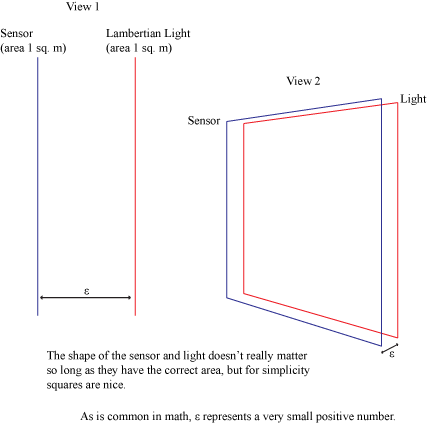## Monte Carlo Forward/Backward Radiance in Basic Example

Practical and theoretical implementation discussion.
Geometrian
Posts: 29
Joined: Sun Jul 28, 2013 6:53 pm
Contact:

### Monte Carlo Forward/Backward Radiance in Basic Example

I have the following simple example:
--Sensor: 1 square meter panel, records radiant flux ϕ
--Light: 1 square meter panel, Lambertian (constant radiance) emission of L=1.0.
--The sensor and light are directly adjacent to each other. You can think of this a sensor plate and a light plate stuck together so that there is no space between.
--Notice that, because of this, all light leaving from one side of the light is absorbed by the sensor.

By integration, I can find that the total radiant flux the plate emits is 2πL, which is just πL for one side. Therefore, computation of the Monte Carlo estimator for both forward and backward directions should result in an expected value of ϕ=πL for the sensor readout.

Monte Carlo estimator:
E[g(x)] ≈ g(x_i)/pdf(x_i)

By path tracing (forward; starting a ray from the sensor):
--The integral is the conversion from radiance L into radiant flux ϕ, which can be derived from the definition of radiance. In the below, theta is the angle between w and the sensor's normal.
--Integral: ϕ=int_area int_sphere L(w)*|cos(theta)|*dw*da
--Monte Carlo g: g(w)=L(w)*|cos(theta)| <-- note L(w) is zero for half the directions
--Monte Carlo pdf: pdf(w)=1/(4π) * 1/1 <-- the ray is chosen randomly from the sphere, and randomly from the area
--Monte Carlo estimate: E[ϕ]=E[g(w)]/E[pdf(w)]=(0.25L)/(1/(4π))=πL <-- agrees with the value above.

By light tracing (backward; starting a ray from the light):
--I feel like there should be symmetry here, but I haven't been able to exploit it.
--I expect the integral is as above
--Every attempt I have made fails to get the same answer. Can anyone show me what the correct Monte Carlo g, pdf, and resulting estimate are?

Thanks,
-G

ingenious
Posts: 282
Joined: Mon Nov 28, 2011 11:11 pm
Location: London, UK
Contact:

### Re: Monte Carlo Forward/Backward Radiance in Basic Example

I'm having troubles picturing your scene setup. Can you post a simple drawing?

Geometrian
Posts: 29
Joined: Sun Jul 28, 2013 6:53 pm
Contact:

### Re: Monte Carlo Forward/Backward Radiance in Basic Example

It's a very simple scene:ingenious
Posts: 282
Joined: Mon Nov 28, 2011 11:11 pm
Location: London, UK
Contact:

### Re: Monte Carlo Forward/Backward Radiance in Basic Example

Thanks for the figure. There is symmetry indeed, but you need to define your problem properly. What you want to compute is the total incident power (flux) on the sensor from the light. So you need to integrate the irradiance at every point on the sensor. The irradiance at a point is given as the integral over the hemisphere of the incident radiance times the cosine with the normal. So you get a double integral for the total power on the sensor, as you've written above. To make the symmetry obvious, you can change the irradiance integral to be over the area of the light, not incident directions. Then you have a double integral over the areas of both surfaces, and path and light tracing only differ in the sampling technique they use to generate the two points. Path tracing generates a point on the sensor and then samples the point on the light via a random direction form the sensor point. Light tracing does the opposite. Both estimate that same integral.

I would have liked to write this with the formulas, but sadly we don't have LaTeX support in this forum.

Geometrian
Posts: 29
Joined: Sun Jul 28, 2013 6:53 pm
Contact:

### Re: Monte Carlo Forward/Backward Radiance in Basic Example

I think I understand it now. It's literally exactly the same integral, just with the role of emitter and collector switched. Just as there is no extra cosine weighting needed when an eye-traced ray accumulates radiance from a light, there is no extra cosine weighting needed when a light-traced ray accumulates importance from the sensor.

After some minor fixes, I am now getting correct results.

Thanks,